# Diffusion Model for Simulating the Kinetics of Boronizing Process in the Case of FeB/Fe2B Bilayer Configuration

Diffusion Model for Simulating the Kinetics of Boronizing Process in the Case of FeB/Fe2B Bilayer Configuration

Omar Belguendouz* Bendaoud Mebarek Mourad Keddam Yassine El Guerri

LSCT Synthesis and Catalysis Laboratory, University of Tiaret, Tiaret 14000, Algeria

The Research Laboratory of Industrial Technologies, University of Tiaret, Tiaret 14000, Algeria

Laboratoire de Technologie des Matériaux, Faculté de Génie Mécanique et Génie des Procédés, USTHB, B.P No. 32, 16111, El-Alia, Bab-Ezzouar, Algiers, Algeria

Corresponding Author Email:
omar.belguendouz@univ-tiaret.dz
Page:
191-197
|
DOI:
https://doi.org/10.18280/acsm.440306
25 October 2019
|
Accepted:
11 May 2020
|
Published:
20 August 2020
| Citation

OPEN ACCESS

Abstract:

In this work, we developed a diffusion model based on the second Fick’s law and the solving of the mass balance equations of the (FeB/Fe2B) interface to simulate the boronizing kinetics in the case of bilayer configuration (FeB/Fe2B) formed on AISI D2 steel. It is known that the boronizing process is a thermochemical surface treatment generally carried out at temperatures ranging between 1223 K and 1323 K. The knowledge of the temperature and the processing time are necessary to simulate and optimize the boronizing process. The developed model in this study is used to estimate the value of the growth rate constant in each phase, to simulate the boride layer thickness formed on AISI D2 steel, to determine the boron concentration profile and to evaluate the mass gain at the surface of the borided AISI D2 steel. To validate the developed model, we used the experimental data taken from the literature concerning the layers thicknesses of FeB and Fe2B layers obtained for different process parameters. Finally, these experimental values are compared to the calculated results. A good agreement was observed between the simulated results and the experimental data.

Keywords:

boronizing, simulation, iron borides, diffusion model, fick’s law

1. Introduction

Boronizing is a thermochemical treatment in which the boron atoms are diffused into the surface of a treated material at high temperatures generally between 1223 K and 1373 K [1, 2], with an aim of forming a boronized layer, the diffused boron atoms in the iron matrix lead to the formation of two kinds of iron (FeB and Fe2B)in case of steels or Armco iron , the formed boronized layer confers to the material a high surface hardness (>1700Hv) which is maintained at high temperatures . This thermochemical treatment provides to the material a good resistance to abrasive and adhesive wear.

Boronizing can be carried out by different methods. The medium of boronizing can be solid (paste or powder), liquid or gas [3, 4].

With the use of this treatment and based on many studies, for a low potential we have only the formation of a single boronized layer constituted of Fe2B phase. In the case of a higher activity of boron, we have a bilayer configuration (FeB +Fe2B) [5, 6]. From the experimental standpoint, the Fe2B and FeB layers have a saw-toothed morphology in case of carbon steels, low alloy steels and Armco iron .

Generally, in the industrial applications, a single layer Fe2B is preferred because it is less brittle and forms a surface having a high compressive stress.

The bilayer configuration (FeB+Fe2B) can be converted into a single layer (Fe2B) by the diffusion annealing process [1-7] to improve its surface properties.

The quality of the boronized layer depends on the chemical composition of both, the base material and the boronizing medium [7, 8].

Generally, the presence of some alloying elements in the treated material modifies strongly the structure of the boride layer by diminishing its thickness and slowing down the diffusion rate of boron atoms [1-9].

For the boronizing in powders, the samples are placed in the container filled with powders mixture containing a boron source, an activator and a diluent and they are put into the muffle furnace.

The significant advantages of this process are easy handling, simplicity, and the possibility of changing the chemical composition of the powder [7-10].

We can use the liquid boronizing process in molten salts with or without electrolysis.

In the gaseous medium, the gases supplying boron are boron halides (diborane and trimethyl) .

The growth kinetics of boride layers requires a mathematical model to simulate the boron diffusion and to calculate the boronized layer thickness in each phase.

In the literature, there are various models [2, 11] used for modeling the boronizing kinetics of steels. Some of these models allow modeling the growth kinetics of Fe2B and/or FeB layers. Furthermore, the majority of these models are based on the mass balance equations at the considered interfaces.

Theoretically, Brakman et al. , have proposed a simple model to study the boronizing process in powders. This model accounts for the specific difference in volume between the FeB and Fe2B phases.

Zuno-Silva et al.  and Campos et al.  have suggested a mathematical model based on the second Fick’s law to study the kinetics of the single layer (Fe2B). Recently, Keddam et al.  proposed a diffusion model basing on the integral method to investigate the boronizing kinetics of AISI D2 steel. This model takes also into account the incubation times during the formation of boronized layers. A diffusion model was proposed by Mebarek et al.  in order to calculate the boride incubation time.

Campos et al. , Mebarek et al. and Genel et al. [9-17] applied the artificial intelligent method for modeling the boronizing kinetics.

In other model [12, 18], a fuzzy neural and neural network was used as an alternative approach for predicting the values of boronized layer thickness. This approach considered, as a simple tool, was employed to improve the previous model (the artificial network model) by using a few conditions to resolve the kinetics equations.

Recently, the LS-SVM  and the Neural-Fuzzy  methods were also used for estimating the boronizing kinetics. These two approaches were able to predict in a satisfactory way the boronized layer thickness.

In this study, a mathematical model of the boron diffusion was suggested to simulate the boronizing kinetics of AISI D2 steel in the case of powder method. The present diffusion model was based on solving the mass balance equations at the two growing interfaces (FeB/Fe2B) and (Fe2B) by ignoring the effect of boride incubation times on the kinetics.

The proposed model considered that the boron concentration profile was nonlinear in each phase. The influence of surface boron concentration on the growth kinetics of FeB and Fe2B layers was investigated. The mass gain associated with the formation of iron borides was also estimated.

Finally, the present model has been validated experimentally by comparing the boride layers’thicknesses with the predicted ones.

2. Mathematical Model of the Diffusion

Based on the second Fick’s law and the mass balance equations, we developed a model to simulate the kinetics of the bilayer configuration.

The knowledge of thermodynamic properties of the Fe-B phase diagram is significant for the boronizing process of AISI D2 steels and associated ferrous materials.

To solve a problem of the boron diffusion, the knowledge of the kinetic data and thermodynamic data are necessary.

From the Fe-B phase diagram, we note the existence of the following phases,a-Fe for the temperature range T£1184.6 K, g-Fe, FeB and Fe2B.

Moreover, it can be seen that the solubility of boron in the phase g-Fe is extremely low at high temperatures (T³1184.6 K).

The diffusion of boron in the iron matrix can be described with the Fick’s second law.

$\frac{\partial C_{i}}{\partial t}=D_{i} \frac{\partial^{2} C_{i}(x, t)}{\partial x^{2}}$      (1)

With

Ci(x,t): is the boron concentration in-depth x after a time t of diffusion.

Di: is the boron diffusion coefficient in phase i, with i = (FeB, Fe2B or Fe).

Figure 1 illustrates a schematic representation of the variation of the boron concentration at a given temperature and under a high boron potential which allows obtaining the two-phase configuration (FeB and Fe2B).

## 1.pngFigure 1. Schematic representation of the boron concentration profile through each boride layer 

The present diffusion model for studying the growth kinetics of FeB and Fe2B considered that:

• The flux of boron atoms is perpendicular to the material surface.
• The temperature of the sample is constant during the process.
• The boron concentration at the material surface does not change with the time and the treatment temperature.
• The iron borides grow according to a parabolic law of time.
• The boride layer is very thin compared to the thickness of the sample.
• The diffusion of Fe can be neglected.

To calculate the shift of the interface (FeB/Fe2B) and (Fe2B/Fe), the masse balance equations given by the following formulas are used:

$\left(\frac{1}{2}\left(C_{s}-C^{F e / F e_{2} B}\right)+\Delta C_{1}\right) \frac{d \lambda_{1}}{d t}=\left(\Delta j_{a}\right)_{x=\lambda_{1}}$     (2)

$\left(\frac{1}{2} \Delta C_{2}+\Delta C_{3}\right) \frac{d \lambda_{2}}{d t}+\frac{\Delta C_{2}}{2} \cdot \frac{d \lambda_{2}}{d t}=\left(\Delta j_{b}\right)_{x=\lambda_{2}}$      (3)

With:

$\Delta C_{1}=C^{F e_{2} B / F e B}-C^{F e B / F e_{2} B}$

$\Delta C_{2}=C^{F e B / F e_{2} B}-C^{F e / F e_{2} B}, \Delta C_{3}=C^{F e / F e_{2} B}-C^{F e_{2} B / F e}$

$\Delta J_{a}=J_{1}-J_{2}, \Delta j_{b}=J_{3}-J_{2}$   with (1=FeB, 2=Fe2B, 3=Fe)

The flux Ji of the boron atoms in the phase i with depth x which is related to the concentration gradient is given by Eq. (4):

$J_{i}=-D_{i}\left(\frac{\partial C_{i}(x, t)}{\partial x}\right)$     (4)

The thickness of the boronized layer is given by the following equation:With i = (FeB, Fe2B, Fe) and Di the associated boron diffusion coefficient.

$\lambda_{F e B}=k_{F e B} \sqrt{t}$ and $\lambda_{F e_{2} B}=k_{F e_{2} B} \sqrt{t}$     (5)

In Eq. (5), $\mathrm{k}_{\mathrm{FeB}}$ and $k_{F e_{2} B}$  are the respective values of growth rate constants for FeB and Fe2B phases. $\mathrm{k}_{\mathrm{FeB}}$ and $k_{F e_{2} B}$  represent the positions of the interfaces (FeB/Fe2B) and (Fe/Fe2B) respectively.

The growth rate constant can be calculated from the resolution of the nonlinear Eqns. (2) and (3) by means of the Newton-Raphson numerical method.

The boron concentration in the iron matrix during the boronizing is given by Eq. (6). This formula represents a general solution of the second Fick’s law (1):

$C_{i}(x, t)=a_{i}+b_{i} \operatorname{erf}\left(\frac{x}{2 \sqrt{D_{i} t}}\right)$     (6)

Ci(x, t): is the boron concentration in the iron matrix depending on the time and diffusion distance x where i denotes the iron phase.

where, erf is a Gauss error function, ai and bare the constants to be determined according to the initial conditions and boundary conditions:

Initial condition:

for t=0 and x>0, C(x, 0)=0

Boundary condition (t>0):

- for x=0,

$C_{F e B}(0, t)=C_{B}^{S / F e B}$ (the boron concentration at the surface).

- At the (FeB/Fe2B) interface:

for x = $\lambda_{F e B}$

$C_{F e B}\left(\lambda_{F e B}, t\right)=C_{B}^{F e_{2} B / F e B} \quad C_{F e_{2} B}\left(\lambda_{F e B}, t\right)=C_{B}^{F e B / F e_{2} B}$

At the (Fe2B/Fe) interface:

for x = $=\lambda_{F e_{2} B}$

$C_{F e_{2} B}\left(\lambda_{F e_{2} B}, t\right)=C_{B}^{F e / F e_{2} B} \quad C_{F e}\left(\lambda_{F e_{2} B}, t\right)=C_{B}^{F e_{2} B / F e}$

for x = $\mathrm{x}=\infty, \quad C_{F e}(\infty, t)=0$

For our simulation, we used the input data, the diffusion coefficient in each phase, the surface boron concentration and boron concentrations at the considered interfaces.

The boron diffusion coefficients in the iron borides and the Fe phase were taken from references [2, 20] which are given by the following expressions:

$D_{B}^{F e B}=1.7151 \times 10^{-4} \exp \left(-\frac{187.24 \times 10^{3}}{R T}\right)$     (7)

$D_{B}^{F e_{2} B}=8.6579 \times 10^{-5} \exp \left(-\frac{177.39 \times 10^{3}}{R T}\right)$     (8)

For 1184.6 K ≤ T ≤ 1273 K:

$D_{B}^{\gamma-F e}=4.4 \times 10^{-8} \exp \left(-\frac{81.5 \times 10^{3}}{R T}\right)$    (9)

For T<1184.6 K:

$D_{B}^{\alpha-F e}=8.3 \times 10^{-9} \exp \left(-\frac{62.7 \times 10^{3}}{R T}\right)$     (10)

With R=8.314 J/mol K

The value of the boron concentration at the surface used in the simulation is Cs=16.48(wt%). For the two interfaces (FeB/Fe2B) and (Fe2B/Fe), we used the values taken from the literatures [20, 21] which are the following:

$C_{B}^{F e_{2} B / F e B}=16.23 \mathrm{wt} . \%, C_{B}^{F e B / F e_{2} B}=16.23 \mathrm{wt} . \%$,

And

$C_{B}^{F e / F e_{2} B}=8.83 w t . \% ; C_{B}^{F e_{2} B B / F e}=35 \times 10^{-4} w t . \%$.

3. Experimental Validation of the Model

The validation of the present model is based on the experimental data provided by Campos-Silva et al. .

In their experimental work, the AISI D2 steel was used as the substrate to be boronized, its chemical composition is depicted in Table 1.

Table 1. Chemical composition of AISI D2 steel

 % C % Si % Mn % Cr % Mo % V 1.40-1.60 0.10-0.60 0.10-0.60 11.0-13.0 0.70-1.20 0.50-1.10

The boride layers were produced by applying the powder-pack boronizing in the range of 1223 K to 1273 K for different treatment times.

The thickness determined in their experiments for the boronized layers was the average of many measurements made in various points on the treated samples.

4. Results and Discussions

A computer code was written in Matlab R2015b to simulate the boronizing kinetics. The outputs of our program are the growth rate constant for each phase, the boride layer thickness and the boron concentration in each phase (Fe2B, FeB and Fe).

The data collected from the previous section were used as an input data for the computer simulation program, the kinetics data and boron activation energies for FeB and Fe2B in AISI D2 steel were taken from the reference . Boron diffusion coefficients in the α-Fe and g-Fe phase were found in the paper .

4.1 Growth rate constant calculation

Figure 2 describes the temperature dependence of growth rate constant at each interface during the growth of bilayer (FeB/Fe2B) on AISI D2 steel in the temperature range of 1223-1273 K.

According to Figure 2, the growth rate constant in each phase (FeB and Fe2B) follows an exponential law as a function of the boriding temperature.

A good agreement is noted between the experimental data and the simulation results.

## 2.png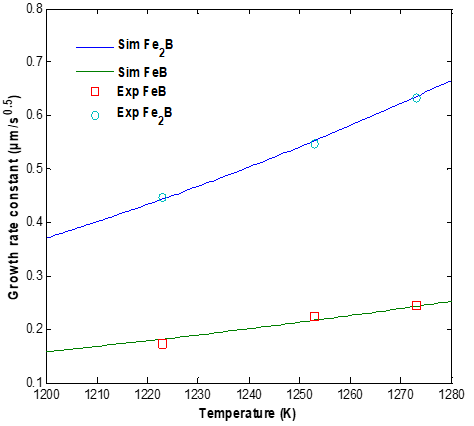Figure 2. Variation of the growth rate constant in each phase versus the boriding temperature

4.2 The boronized layer thickness

Figures 3 and 4 show the evolution of the square of layers thicknesses of FeB and Fe2B layers grown at the surface of AISI D2 steel as a function of treatment time.

At first sight, it can be seen that the boronized layer thickness develops according to the parabolic law and the simulated curves coincide with the experimental data.

## 3.png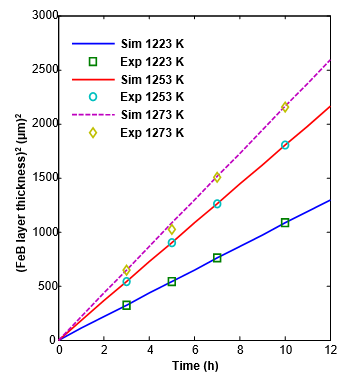Figure 3. Evolution of square of FeB layer thickness as a function of treatment time

4.3 Effect of boron concentration on the boride layer thickness

We calculate the growth rate constant by varying the boron concentration at the material surface. The growth kinetics of the boronized layer in each phase depends on the value of surface boron concentration and the treatment temperature. It is noticed that the increase in the temperature of the process activated the phenomenon of boron diffusion inside the material.

Figure 5 and Figure 6 depicted the variation of the growth rate constant as a function of the surface boron concentration and we get from this result that the growth rate constant in each phase depends on the boron concentration and the process temperature.

In the case of the FeB layer, we note that the growth rate constant increases with the increasing of the temperature and the boron concentration at the material surface.

For the Fe2B layer, it is seen that its growth rate constant is decreased when increasing the value of surface boron concentration at a given boriding temperature.

This is interpreted by the augmentation of the layer thickness of FeB which corresponds to the decrease in the Fe2B boride layer thickness.

From Figures 5 and 6, it is demonstrated that the surface boron concentration exerts a strong influence on the kinetics of FeB layer and less for the Fe2B layer.

## 4.pngFigure 4. Evolution of square of Fe2B layer thickness as a function of treatment time

## 5.png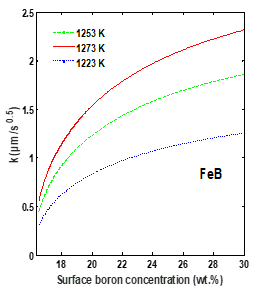Figure 5. Growth rate constant according to the temperature and the surface boron concentration for the FeB layer

## 6.png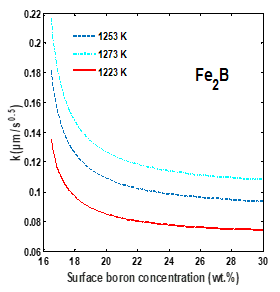Figure 6. Growth rate constant according to the temperature and the surface boron concentration for the Fe2B layer

4.4 Boron concentration for each phase

The developed computer simulation program allows us to follow the boron distribution through each boride layer.

To calculate the boron concentration in the Fe2B and FeB phases, we used the following equations while considering the initial and the boundary conditions:

for $0 \leq x \leq \lambda_{F e B}$

$C_{F e B}(x, t)=$

$C_{B}^{S / F e B}+\frac{C_{B}^{F e B / F e_{2} B}-C_{B}^{S / F e B}}{\operatorname{erf}\left(\frac{\lambda_{F e B}}{2 \sqrt{D_{B}^{F e B}}}\right)} \times \operatorname{erf}\left(\frac{x}{2 \sqrt{D_{B}^{F e B} t}}\right)$      (11)

for $\lambda_{F e B} \leq x \leq \lambda_{F e_{2} B}$

$C_{B}^{F e B / F e_{2} B}+\frac{C_{B}^{F e_{2} B / F e}-C_{B}^{F e B / F e_{2} B}}{\operatorname{erf}\left(\frac{\lambda_{F e B}}{2 \sqrt{D_{B}^{F e_{2} B}} t}\right)-\operatorname{erf}\left(\frac{\lambda_{F e_{2} B}}{2 \sqrt{D_{B}^{F e_{2} B}} t}\right)}$  ×   $\left[\operatorname{erf}\left(\frac{\lambda_{F e B}}{2 \sqrt{D_{B}^{F e_{2} B}} t}\right)-\operatorname{erf}\left(\frac{x}{2 \sqrt{D_{B}^{F e_{2} B}} t}\right)\right]$       (12)

Figures 7, 8 and 9 depicted the variation of the boron concentration in different phases: FeB, Fe2B and Fe, the concentration of boron decreases gradually according to the penetration depth.

It is seen that the time duration plays an important role during the evolution of growth kinetics of FeB and Fe2B layers formed at the surface of AISI D2 steel.

To estimate the mass gain, we used the Eqns. 13 and 14, the calculation is based on the assumptions that the FeB and Fe2B layer forms instantaneously and immediately covers the specimen surface:

$G(t)_{F e B}=\frac{2 p\left(C_{B}^{S / F e B}-C_{B}^{F e B / F e_{2} B}\right)}{\operatorname{erf}\left(\frac{k_{F e B}}{2 \sqrt{D_{B}^{F e B}}}\right)} \sqrt{\frac{D_{B}^{F e B} t}{\pi}}$      (13)

For the estimation of the mass gain associated to Fe2B phase we used the following equation:

$G(t)_{F e_{2} B}=\frac{2 p\left(C_{B}^{F e_{2} B / F e}-C_{B}^{F e_{2} B / F e B}\right)}{\operatorname{erf}\left(\frac{k_{F e B}}{2 \sqrt{D_{B}^{F e_{2} B}}}\right)-\operatorname{erf}\left(\frac{k_{F e_{2} B}}{2 \sqrt{D_{B}^{F e_{2} B}}}\right)} \sqrt{\frac{D_{B}^{F e_{2} B_{t}}}{\pi}}$      (14)

With:

$G_{F e B}$ and $G_{F e_{2} B}$  are the mass gain per unit surface of FeB and Fe2B phase.

$\rho$ is the specific volume of pure iron (=7.86 g.cm–3).

## 7.png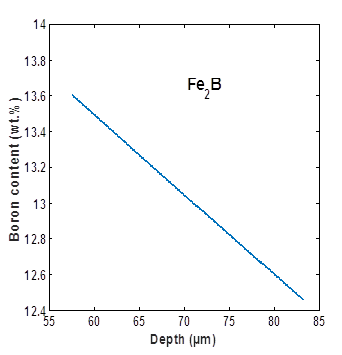Figure 7. Boron concentration in the Fe2B phase for T=1172 K and surface boron surface concentration of 16.48 wt.%

## 8.png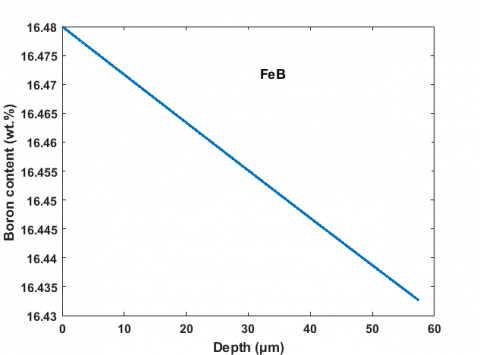Figure 8. Boron concentration in the FeB phase for T=1172 K and surface boron surface concentration of 16.48 wt.%

## 9.pngFigure 9. Boron concentration in the a-Fe phase for T=1172 K and surface boron surface concentration of 16.48 wt.%

## 10.png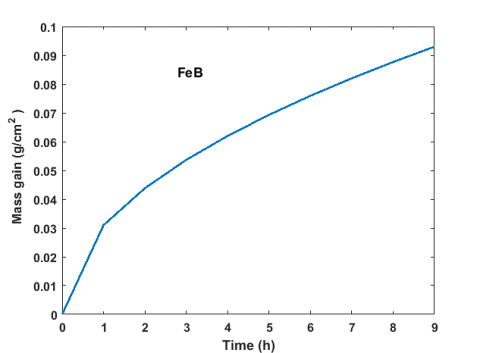Figure 10. Mass gain in the FeB phase calculated at T=1172 K with surface boron concentration of 16.48 wt.%

## 11.png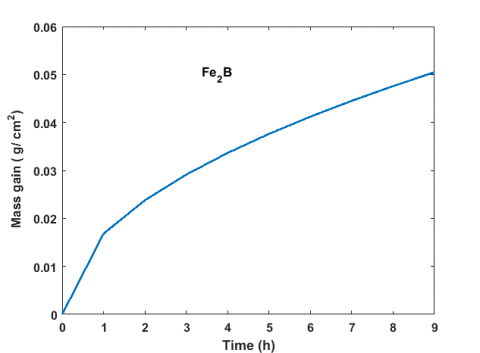Figure 11. Mass gain in the Fe2B phase calculated at T=1172 K with surface boron concentration of 16.48 wt.%

Figures 10 and 11 depicted the variation of the mass gain in FeB and Fe2B phase; the mass gain generated by the boronizing process on AISI D2 steel followed a parabolic law. It is noticed that the calculated values of mass gain at the material surface are affected by the change in the time duration for a given boronizing temperature.

The mass gain associated with the formation of each boride layer is significantly increased with the process temperature due to a high mobility of boron in each boride layer.

5. Conclusion

In this work, a diffusion model based on the Fick second law and the mass balance equations at the two growing interfaces (FeB/Fe2B) and (Fe2B/Fe) was considered.

It was applied to investigate the kinetics of formation of FeB and Fe2B layers on AISI D2 steel in the temperature range of 1223-1273 K.

The created computer program was able to calculate the boronized layer thickness, estimate the boron concentration in each phase and calculate the mass gain in each phase.

The formation rates of the Fe2B and FeB layers had a parabolic character for all temperatures.

The developed diffusion model has been validated by using the experimental data provided by Campos et al. .

A good agreement was then noticed between the experimental data and the simulation results.

Moreover, the surface boron concentration had a strong influence on the growth of FeB layer; nevertheless, this effect was less pronounced for the Fe2B layer. The estimated values of mass gain at the material surface were greatly affected by the time duration because of a high mobility of boron in each boride layer (FeB and Fe2B) at a given temperature.

Finally, the modeling of the growth kinetics of boronized layer can provide a simple tool to study the influence of process parameters on the diffusion rate of boron atoms at the surface of AISI D2 steel.

References

 Sinha, A.K. (1991). Boriding (Boronising). Journal of Heat Treating, 4: 437-446.

 Keddam, M., Kulka, M. (2018). Simulation of the growth kinetics of FeB and Fe2B layers on AISI D2 steel by the integral method. Physics of Metals and Metallography, 119(9): 842-851. https://doi.org/10.1134/S0031918X18090065

 Brakman, C.M., Gommers, A.W., Mittemeijer, E.J. (1989). Boriding of Fe and Fe–C, Fe–Cr, and Fe–Ni alloys; Boride-layer growth kinetics. Journal of Materials Research, 4(6): 1354-1370. https://doi.org/10.1557/JMR.1989.1354

 Nam, K.S., Lee, K.H., Lee, D.Y., Song, Y.S. (2005). Metal surface modification by plasma boronizing in a two-temperature-stage process. Surface and Coatings Technology, 197(1): 51-55. https://doi.org/10.1016/j.surfcoat.2004.10.099

 Ortiz-Domínguez, M., Elias-Espinosa, M., Keddam, M., Gómez-Vargas, O.A., Lewis, R., Vera-Cárdenas, E., Zuno-Silva, J. (2015). Growth kinetics and mechanical properties of Fe2B layers formed on AISI D2 steel. Indian Journal of Engineering and Materials Sciences, 22(2): 231-243.

 Ortiz-Domínguez, M., Keddam, M., Elias-Espinosa, M., Damián-Mejía, O., Flores-Rentería, M.A., Arenas-Flores, A., Hernández-Ávila, J. (2014). Investigation of boriding kinetics of AISI D2 steel. Journal of Surface Engineering, 30(7): 490-497. https://doi.org/10.1179/1743294414Y.0000000273

 Graf von Matuschka, A. (1980). Boronizing. Carl Hanser Verlag, Munich, FRG.

 Melendez, E., Campos, I., Rocha, E., Barron, M.A. (1997). Structural and strength characterization of steels subjected to boriding thermochemical process. Materials Science and Engineering A, 234-236: 900-903. https://doi.org/10.1016/S0921-5093(97)00389-4

 Mebarek, B., Zanoun, A., Rais, A. (2016). Comparaison de deux approches numériques pour le traitement de boruration thermochimique de l’acier XC38. Metallurgical Research & Technology, 113(1): 104-114. https://doi.org/10.1051/metal/2015046

 Kulka, M. (2019), Current Trends in Boriding Techniques. Engineering Materials book series, Springer International Publishing.

 Mebarek, B., Madouri, D., Zanoun, A., Belaidi, A., (2015). Simulation model of monolayer growth kinetics of Fe2B phase. Matériaux & Techniques, 103(7): 703-709. https://doi.org/10.1051/mattech/2015058

 Mebarek, B., Keddam, M. (2019). Prediction model for studying the growth kinetics of Fe2B boride layers during boronizing. Ingénierie des Systèmes d’Information, 24(2): 201-205. https://doi.org/10.18280/isi.240212

 Zuno-Silv, J., Ortiz-Domínguez, M., Keddam, M., Elias-Espinosa, M., Damián-Mejía, O., Cardoso-Legorreta, E., Abreu-Quijano, M. (2015). Boriding kinetics of Fe2B layers formed on AISI 1045 steel. Journal of Mining and Metallurgy Section B Metallurgy, 50(2): 101-107. https://doi.org/10.2298/JMMB140323019Z

 Campos, I., Islas, M., González, E., Ponce, P., Ramírez, G. (2006). Use of fuzzy logic for modeling the growth of Fe2B boride layers during boronizing. Surface and Coatings Technology, 201(6): 2717-2723. https://doi.org/10.1016/j.surfcoat.2006.05.016

 Mebarek, B., Keddam, M., Boussebha, H. (2019). Simulation model of the growth kinetics of Fe2B layers with consideration of the boride incubation time effect. Surface Review and Letters, 27(4): 1590127. https://doi.org/10.1142/S0218625X19501270

 Campos, I., Torres, R., Bautista, O., Ramırez. G., Zuniga, L. 2006). Effect of boron paste thickness on the growth kinetics of polyphase boride coatings during the boriding process. Applied Surface Science, 252(6): 2396-2403. https://doi.org/10.1016/j.apsusc.2005.04.022

 Genel, K., Ozbek, I., Kurt, A., Bindal, C. (2002). Boriding response of AISI W1 steel and use of artificial neuronal network for prediction of borided layer proprieties. Surface Coatings Technology, 160(1): 38-43. https://doi.org/10.1016/S0257-8972(02)00400-0

 Mebarek, B., Keddam, M. (2018). A fuzzy neural network approach for modeling the growth kinetics of FeB and Fe2B layers during the boronizing process. Matériaux & Techniques, 106(6): 603-610. https://doi.org/10.1051/mattech/2019002

 Mebarek, B., Keddam, M. Aboshighiba, H. (2018). LS-SVM approach for modeling the growth kinetics of FeB and Fe2B layers formed on Armco iron. Revue des Sciences et Technologie de l’information, 23(5): 29-41. https://doi.org/10.3166/ISI.23.5.29-41

 Keddam, M., Chentouf, S. M. (2005). A diffusion model for describing the bilayer growth (FeB/Fe2B) during the iron powder-pack boriding. Applied Surface Science, 252(2): 393-399. https://doi.org/10.1016/j.apsusc.2005.01.016

 Keddam, M., Nait.Abdellah, Z., Kulka, M., Chegroune, R. (2015). Determination of the diffusion coefficients of boron in the FeB and Fe2B layers formed on AISI D2 steel. Acta Physica Polonica Series A, 128(4): 740-745. https://doi.org/10.12693/APhysPolA.128.740

 Campos-Silva. I., Tadeo-Rosas, R, Santos-Medina, H.D., Lopez-Garcıa, C. (2015). Boride Layers: Growth Kinetics and Mechanical Characterization, Encyclopedia of Iron. Steel, and Their Alloys. Five-Volume Set, Eds. R. Colas, G.E. Totten. https://doi.org/10.1081/E-EISA-120052666